# Lines And Angles - Basic Terms

Lines And Angles are the basic shapes in geometry. Lines are figures that are made up of infinite points extending indefinitely in both directions. Lines are straight and have negligible depth or width. There are a variety of lines you will learn about, such as perpendicular lines, intersecting lines, transversal lines, etc. An angle is a figure in which two rays emerge from a common point. You may also come across alternate and corresponding angles in this field.  Geometry shapes and their properties are the most practical branch of mathematics. Mostly this concept has been taught in Class 7 and Class 9.

## Definition of Lines and Angles

As we have discussed, both lines and angles form the base for any shape in geometry. We cannot draw a two-dimensional to three-dimensional shape without using lines and angles. Thus, it is very necessary to learn the definitions of both terms.

Here, the basic definitions and properties of lines and also for angles are given. It will give the students a basic knowledge of these geometrical terms.

### What are Lines?

A line is a straight one-dimensional figure, that extends in the opposite directions infinitely. A line can be horizontal or vertical. It can be drawn from left to right or top to bottom.

### What are Angles?

Angles are the shape that is formed when the endpoints of two rays meet at a single point. They are measured in degrees (°) or radians. A complete rotation is equal to an angle of 360 degrees. It is represented by the symbol ‘∠’.

## Types of Lines and Angles

There are various types of lines and angles in geometry based on the measurements and different scenarios. Let us learn here all those lines and angles along with their definitions.

### Types of Lines

Lines are basically categorized as:

• Line segment
• Ray

Based on concepts or operations performed on lines, they are;

• Parallel Lines
• Perpendicular Lines
• Transversal

### Line Segment

A line segment is a part of a line with two end-points. It is the shortest distance between two points and has a fixed length.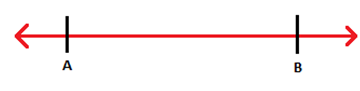### Ray

A ray is a part of a line, which has a starting point and extends infinitely in one direction.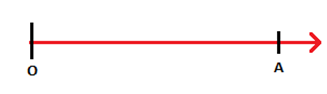### Perpendicular Lines

When two lines form a right angle with each other, by meeting at a single point, are called perpendicular lines. In the figure, you can see, lines AB and CD are perpendicular to each other.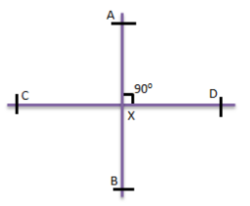### Parallel Lines

Two lines are said to be parallel when they do not meet at any point in a plane or which do not intersects each other. In the figure, lines PQ and RS are parallel to each other.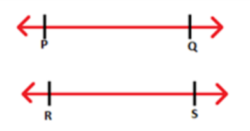### Transversal Line

When a line intersects two lines at distinct points, it is called a transversal. In the figure, a transversal l is intersecting two lines at point P and Q.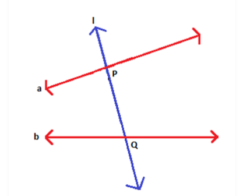### Types of Angles

Angles are basically classified as:

• Acute Angle(<90°)
• Right Angle(=90°)
• Obtuse Angle(>90°)
• Straight Angle(=180°)

And based on the relation between two angles, conceptual wise, they are;

• Supplementary Angles
• Complementary Angles
• Vertically Opposite Angles

### Acute Angle

If the inclination between the arms is less than a right angle, it is called an acute angle.

### Obtuse Angle

If the inclination between the arms is more than a right angle, it is called an obtuse angle.

### Right Angle

If the arms form an angle of 90 degrees between them, it is called a right angle.

### Straight Angle

If the arms form an angle of 180 degrees between them, it is called a straight angle.

### Complementary Angles

Two angles which sum up to 90 degrees are called complementary angles.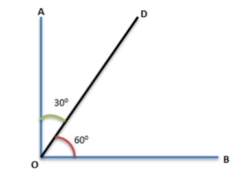### Supplementary Angles

Two angles which sum up to 180 degrees are called supplementary angles.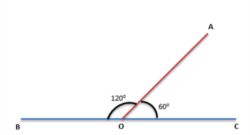Two angles which have a common side and a common vertex are called adjacent angles. In the following figure, ∠α and ∠β are adjacent angles.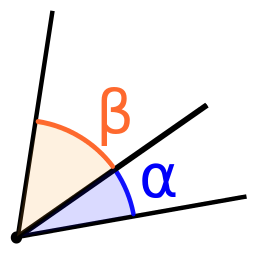### Vertically Opposite Angles

Two angles which are formed, opposite to each other, when two lines intersect at a common point or vertex, are called vertically opposite angles. In the figure, given below;

∠POR = ∠SOQ and ∠POS = ∠ROQ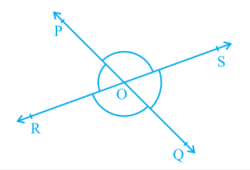## Video Lesson on Lines## Video Lessons on Types and Parts of Angles

### Types of angles### Parts of Angles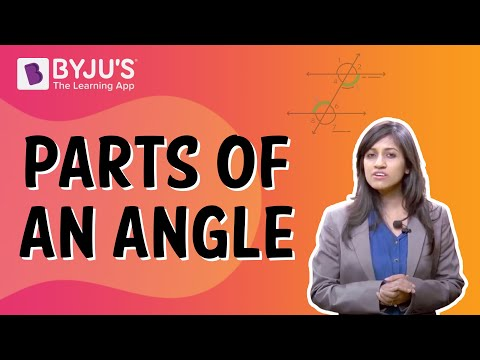## Properties of Lines and Angles

Similar to other shapes and sizes in geometry, lines and angles also have their own properties. Let us see what are they.

### Properties of Lines

• Collinear points are a set of three or more points which lie on the same line.
• The points which do not lie on the same line are called non-collinear points.

Note: Three points can be either collinear or non-collinear, but not both together at the same time.

### Properties of Angles

• An angle is a figure in which two rays emerge from a common point. This point is called the vertex of the angle and the two rays forming the angle are called its arms or sides.
• An angle which is greater than 180 degrees but less than 360 degrees is called a reflex angle.
• If two adjacent angles add up to 180 degrees, they form a linear pair of angles. In the following figure, ∠a and ∠b form a linear pair.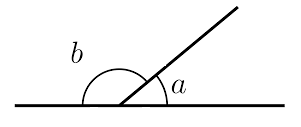• When two lines intersect each other, the two opposite pairs of angles formed are called vertically opposite angles. In the following figure, ∠A and ∠B are vertically opposite angles. Another pair is ∠C and ∠D.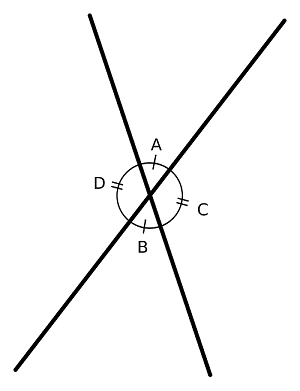## Frequently Asked Questions on Lines And Angles

Q1

### What are the five types of Angles?

The five types of angles are:

1. Acute Angle
2. Right Angle
3. Obtuse Angle
4. Straight Angle
5. Reflex Angle
Q2

### What are the properties of Lines and Angles?

If two parallel lines are intersected by a transversal then:

• Its vertically opposite angles are always equal
• Its corresponding angles are equal
• Its alternate exterior and interior angles are equal
Q3

### What are Lines and its types?

Lines are figures that are made up of infinite points extending indefinitely in both directions. The types of lines are:

1. Horizontal lines
2. Vertical lines
3. Parallel lines
4. Perpendicular lines
Test your knowledge on Lines And Angles

1. Very nice tutors and very useful